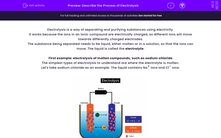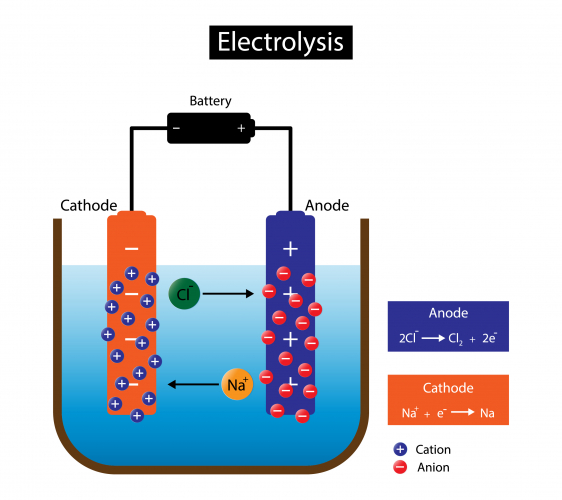# Describe the Process of Electrolysis

In this worksheet, students will study how electricity is used to separate substances in a process called electrolysis. They will also learn to describe electrolysis in terms of electron transfer.Key stage:  KS 4

Year:  GCSE

GCSE Boards:   AQA, AQA Trilogy, AQA Synergy, OCR 21st Century, OCR Gateway, Pearson Edexcel, Eduqas,

Difficulty level:#### Worksheet Overview

Electrolysis is a way of separating and purifying substances using electricity.

It works because the ions in an ionic compound are electrically charged, so different ions will move towards differently charged electrodes.

The substance being separated needs to be liquid, either molten or in a solution, so that the ions can move. The liquid is called the electrolyte.

First example: electrolysis of molten compounds, such as sodium chloride

The simplest types of electrolysis to understand are where the electrolyte is molten.

Let's take sodium chloride as an example. The liquid contains Na+ ions and Cl- ions:Because of the electric current, electrons move through the wires from the anode, through the battery, to the cathode. The anode electrode becomes positively charged, and the cathode electrode becomes negatively charged.

The charges on the anode and cathode then attract the ions in the molten NaCl. The positively charged sodium cations are attracted to the negatively charged cathode because opposite electrical charges attract. The negatively charged chloride anions are attracted to the positively charged anode for the same reason.

Once the ions reach the electrodes, they interact with electrons:

At the anode, the chloride anions lose their excess electrons, and are turned into chlorine atoms. Loss of electrons is called oxidation.

The half-equation for this process is Na+ + e- → Na. We call it a half-equation because this is only half the process, and can't happen by itself.

At the cathode, the sodium cations gain electrons, and are turned into sodium atoms. Gain of electrons is called reduction.

The half-equation for this process is 2Cl- → Cl2 + 2e-.

We can't write Cl- → Cl + e- because chlorine atoms are only found in pairs, not as individual atoms.

The electrons that the chloride loses when it becomes chlorine are equal to the electrons gained by the sodium ions when they become sodium atoms.

Second example: electrolysis of solutions, such as copper sulfate in water

The copper sulfate example is more complicated, but it is a common experiment, so it's useful to know what happens in outline.

The setup is the same as before, but there are now four types of ion in the solution. The copper sulfate gives us Cu2+ and SO42-. The water breaks down into H+ and OH-.

As before, the positive cations are attracted to the cathode and the negative anions are attracted to the anode.

At the anode, the hydroxide anions lose their excess electrons, and the oxygen from the hydroxide becomes oxygen gas. This is oxidation, and the half-equation is 4OH- → O2 + H2O + 4e-.

At the cathode, the copper cations gain electrons, and are turned into copper atoms. Gain of electrons is called reduction. The half-equation is Cu2+ + 2e- → Cu.

If you're wondering what happens to the sulfate - well done, that's a really good question! It's also a tricky question, so you will need to look at the activity on electrolysis of solutions to find out how that works.

Although a lot of the details of electrolysis get complicated - especially once there are more than two ions involved - the main idea is pretty easy.

Melt or dissolve an ionic compound so that the ions can move, then use charged electrodes to pull differently charged ions in different directions.

Are you ready to try some questions now?

### What is EdPlace?

We're your National Curriculum aligned online education content provider helping each child succeed in English, maths and science from year 1 to GCSE. With an EdPlace account you’ll be able to track and measure progress, helping each child achieve their best. We build confidence and attainment by personalising each child’s learning at a level that suits them.

Get started